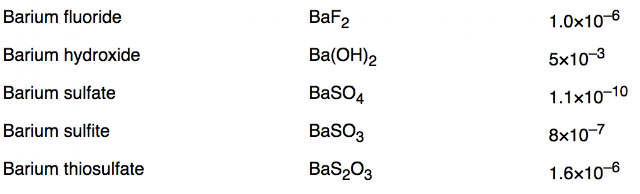### Is BaSO4 soluble or insoluble?

Answer: BaSO4 would typically be considered insoluble due to the presence of a sulfate ion. This usually precludes an ion from disassociating easily in an aqueous solution; this being water.

The idea of BaSO4 being considered insoluble is further borne out in the Ksp values. While these cannot be directly related to molar solubility, they provide a strong hint that Barium sulfate is more stringent than other comparable molecules. For example Ba(OH)2's ksp is approximately seven orders of magnitude less which means that it is much more likely to ionize. You can tell that the molar solubility of barium hydroxide is orders of magnitude greater without performing the necessary calculations. As a reminder a compound is considered insoluble if less than 1% of the compound ionizes.ksp values of some common Barium products (source)

What is the molar solubility of BaSO4?

The calculation is based on the chemical equation for the disassociation of BaSO4 and the formula for ksp (equilibrium constants only include aqueous and gaseous species).

BaSO4 (s) => Ba(2+)(aq) + SO4(2-)(aq)

ksp = [Ba(2+)][SO4(2-)]

Since when we ionize we create the two ions in equal molar quantity we can state the following

[Ba(2+)] = [SO4(2-)] = x

$1.1*10^-10 = x^2$

x = $1.05*10^-5$ M

This concentration indicates that less than 1% of the solution is composed of ionized species.

How does BaSO4 theoretically ionize?

As the equation (BaSO4 (s) => Ba(2+)(aq) + SO4(2-)(aq)) indicates, barium sulfate would disassociate and produce the barium and sulfate ion. In the real world; however, the low molar solubility indicates that very little barium sulfate actually ionizes.